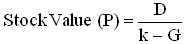# Financial Concept How-To: Gordon Growth Model

The Gordon growth model is widely used for evaluating the intrinsic value of a stock, especially in industries where dividend payments are large and growth is relatively stable. When we take into account the expected annual DPU (dividend per unit), and the assumption that the dividend grows at a constant rate in perpetuity, the model solves for the present value of the infinite series of anticipated dividends. This is just the right financial concept and formula to know in order to quickly evaluate dividend paying counters such as REITs.1. D = Anticipated DPU for the next fiscal year
2. k = Required rate of return
3. G = Dividend growth rate, forever

Dividend growth rate projection in perpetuity is based on historical dividend payouts.

For instance, Kenanga Investment uses the following numbers to value Pavilion REIT at RM 1.13 per share.

D = 5.2 cents,  k = 7.6 percent and G = 3 percent

PAVREIT does not have history of dividend payout, so we don’t really know how is the appropriate rate for dividend growth.  Anyway, is it a coincidence that “G” is equal to Fixed Desposit risk free rate of return?  This does make sense as the dividend payout rate and if possible, the growth rate, must be higher than risk free rate for the investment to be attractive.